Originally published in 2009 at http://www.math.ucla.edu/~rcompton/musical_gauss_seidel/musical_gauss_seidel.html

One second of a 44.1kHz single channel .wav file can be read into an array (call it b) of length 44100. Given a matrix A we seek solutions to the system Ax=b. Through iterations of Gauss-Seidel the vector Ax will approach b with the high frequency parts of Ax getting close to b first. If we take b to be a song recording, some white noise as our initial guess and write out Ax at each iteration we observe that the high pitched notes in b become audible first while at the same time the pitch of the white noise decreases.

Audio of the initial 12 second .wav file (white noise) initialAx.wav

Plots of the initial Ax, residual, and FFT of residual: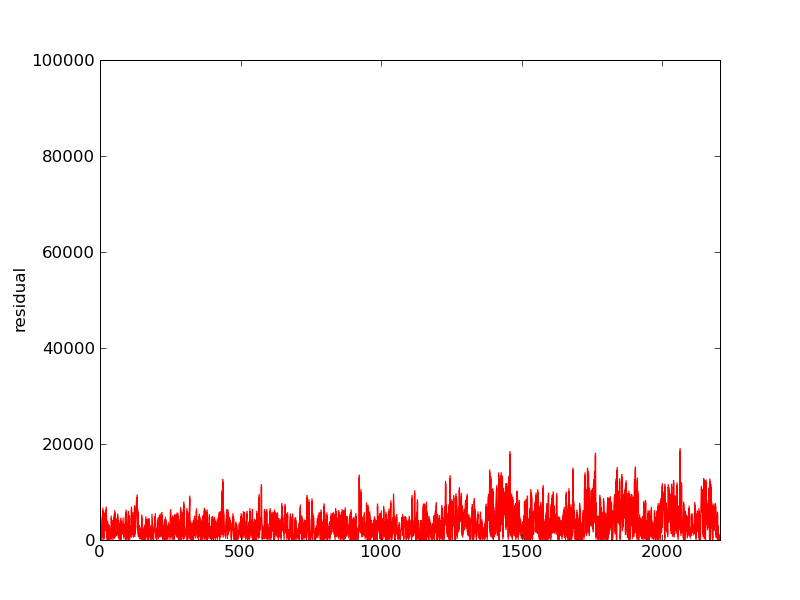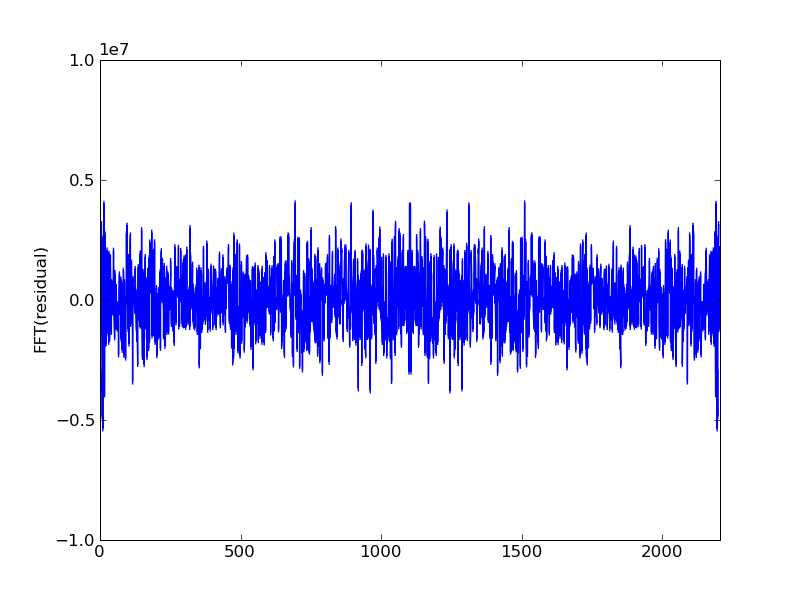After one iteration the high notes become audible gauss_seidel_out000000.wav

And some structure is visible in the spectrum:Second iteration: gauss_seidel_out000001.wav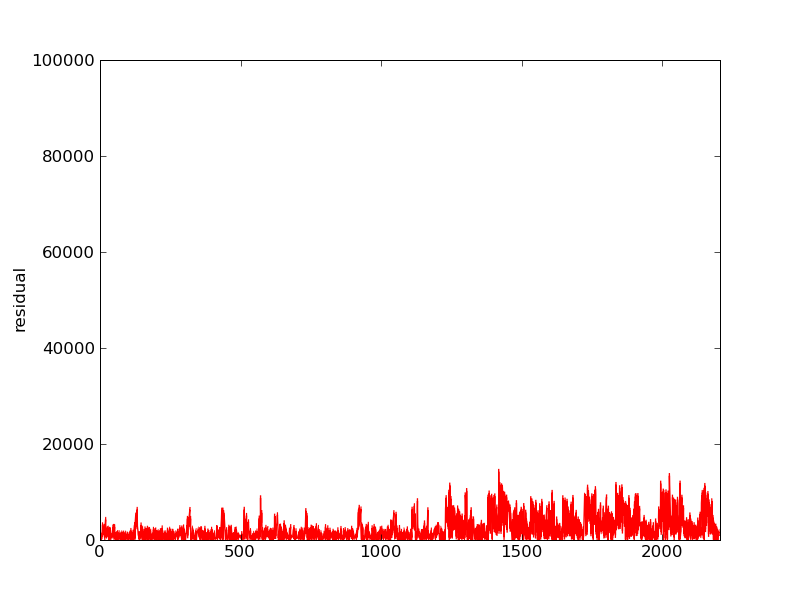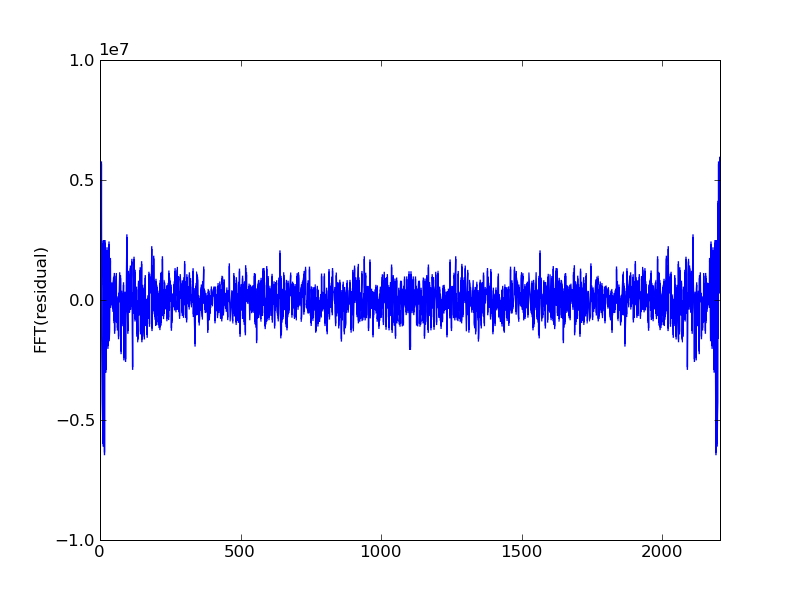Third iteration: gauss_seidel_out000002.wav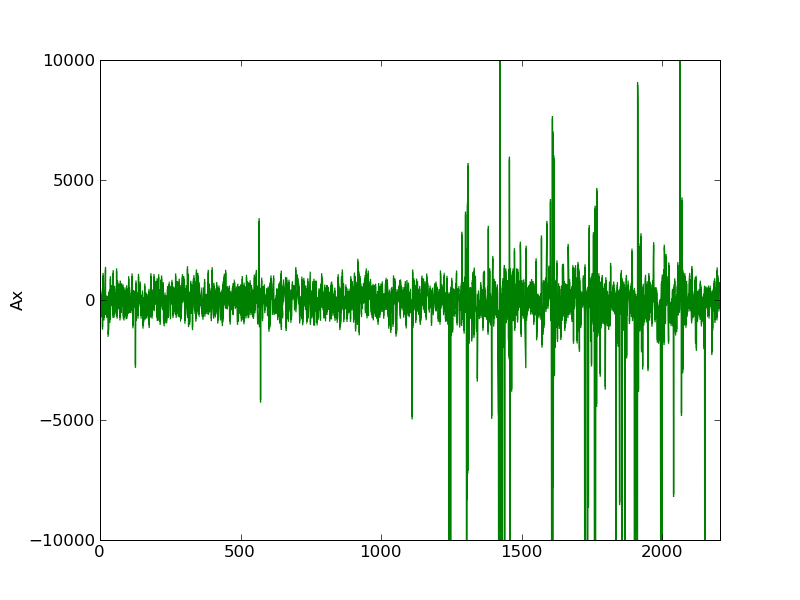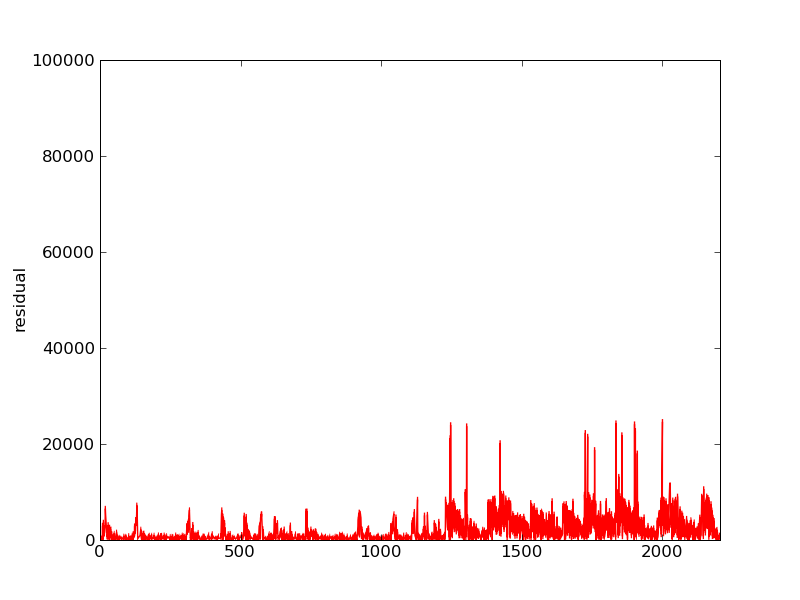Fourth iteration: gauss_seidel_out000003.wav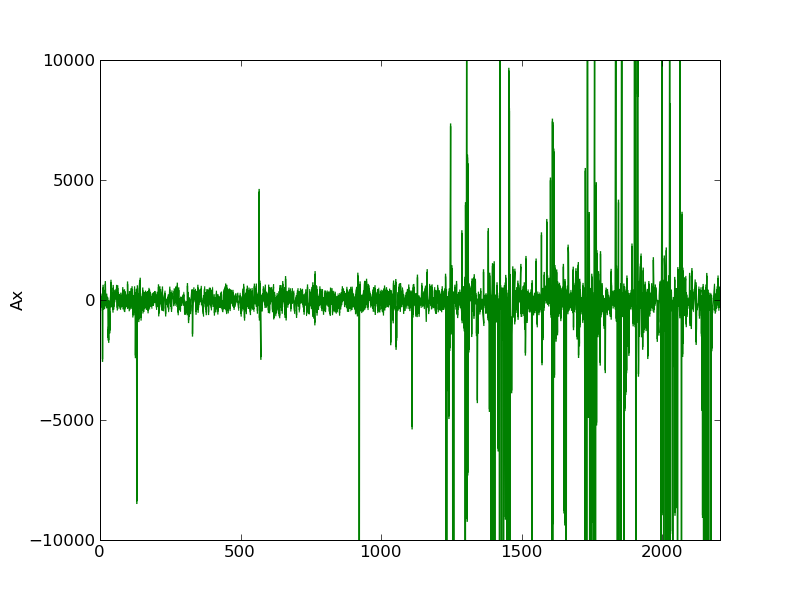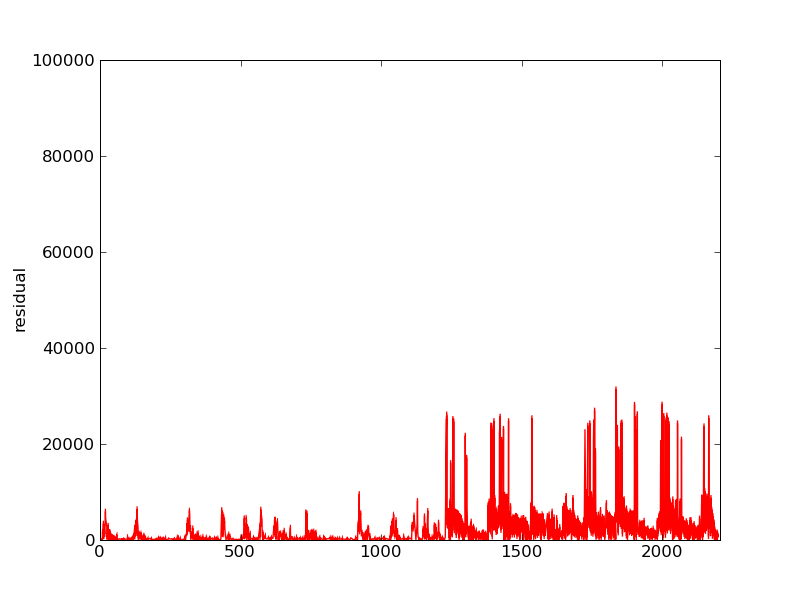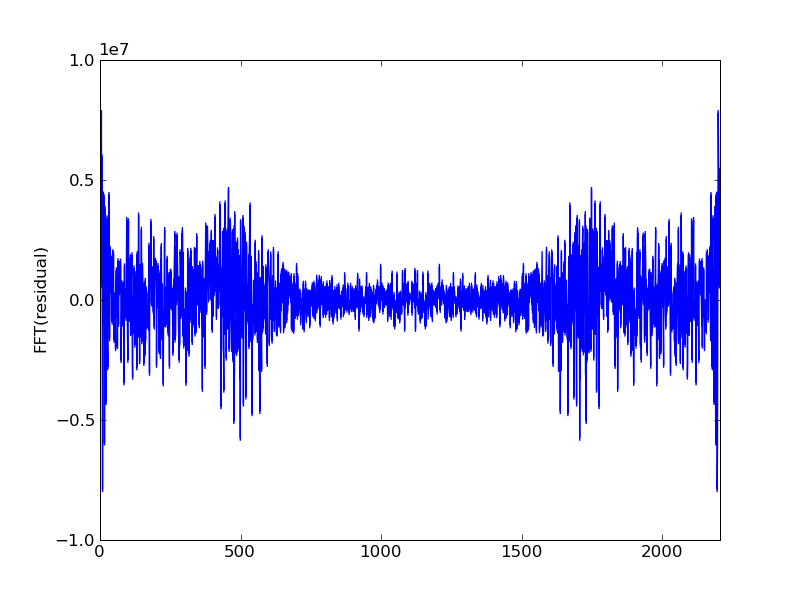This is all done in python. Loading .wav files into arrays is not too bad in scipy. A sparse matrix class is neccessary to avoid memory problems as a 12 second .wav file needs an array of size 12*44100. Here's the TridiagonalMatrix class I used:

And here's the code to handle reading/writing the .wav files and then using Gauss-Seidel to iteratively solve a linear system: